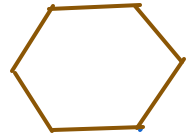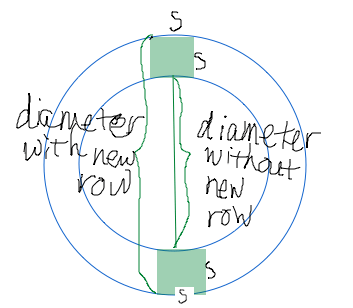## Pi Is for Circles

This post is for crocheters.

## How to crochet circles

Here is how to crochet something round:

Chain three stitches.

1. With a single crochet stitch, connect the last stitch to the first.
2. Single crochet in each of the two remaining chain stitches. You should now have three single crochet stitches at the edge of a tiny circle.
3. To start the next row, keep going in the same direction: Single crochet two stitches in each of the single crochet stitches from the last row. When you finish this row, there should be six stitches on it.
4. For the next row, crochet two single crochet stitches in each of the six single crochet stitches from the last row. Now you should have 12 stitches in the row you just finished.
5. Keep going until your circle is the size you want, increasing 6 stitches with each row, spreading the increases out evenly over the row, placing the increases away from the earlier increases. (If you just keep increasing at the same point on every row, you will get a hexagon.)
6. When you have the size you want, slip stitch the last row. This row has no increases.

There is your circle. A coaster, maybe.

## Why this works

A circle’s circumference, the distance around the circle, equals times the circle’s diameter. “” (a Greek letter, pronounced “pi”) is a number that is equal to about 3.14.

A stitch in single crochet is just about “square,” meaning it’s as high as it is wide. (This is approximate.)

Each stitch increases the circle’s diameter by an amount equal to the distance that the stitch covers in that row of the circle. Let’s call that distance “.”

Each time you complete a row you increase the circle’s diameter by a length of .Since circumference equals times diameter, when you increase diameter by , you also increase circumference by . Each stitch increases the circle’s circumference by a length of , so you need to make each round longer than the one before.

That means you need each row to have 2 more stitches than the row before it has. That’s 6.28 more stitches.

How can you make 0.28 stitches? This is crochet; relax and ignore the 0.28. Then each row needs to have six more stitches than the row before it has.

Note that no matter how big your circle gets, the number of stitches you add to each row does not change. To keep adding rows to the circle just keep adding six stitches per row.

## Interesting aside

Imagine that the Earth is a perfect sphere and you run a thread all the way around it at the equator. Then you lift the thread one inch everywhere. How much additional three do you need to make the end meet the beginning?This is just the crocheted circle extended to the scale of the Earth. To lift the thread all around the planet you need more inches of thread. That’s 6.28 inches of extra thread to lift the rope 1 inch all the way around the Earth.

## Question

Does the sequence converge?

## Solution

A sequence converges if there is a limit for its terms as the number terms goes to infinity.

Is there a limit of as goes to infinity? Let’s see:

. Since the limit as goes to infinity of equals 0, the limit as goes to infinity of .

There is a limit:. Therefore the sequence converges.

### CautionGraph of the 3n/(2n-1). Dots represent sequence values. Value approaches 3/2 as n gets large.

There may be confusion between convergence of a sequence and conversion of a series. Note the distinction: A sequence is a list of numbers: one number, then another number, then another, etc.; while a series is a sum of numbers: one number plus another number, plus another, etc. More confusing, there is a whole topic about convergence of series, with several kinds of tests for convergence.

To determine whether a sequence converges, don’t use the convergence tests that you use for series; instead, look at the limit. While a series converges only if the sum of the terms is limited, a sequence converges if its th term is limited as goes to infinity.

### Update

Paul Headley, who teaches at Northern Virginia Community College, has found an easier way to find the limit: L’Hopital’s rule. L’Hopital’s rule says that if the limits of both the numerator and the denominator of a rational function go to either zero or infinity, then the limit of the rational function is equal to the quotient of the limits of the derivatives of the numerator and the denominator.

In our example: To find the limit as goes to infinity of , start by noting that as goes to infinity, 3n and 2n-1 both go to infinity, so L’Hopital’s rule applies.

Next, replace the numerator and the denominator with their first derivatives, and you get . There’s the limit. Same answer, less sweat.

This post about solving radical equations is part of a series of posts to help you prepare for the Advanced Algebra and Functions part of the Accuplacer test.

## Question

Does the equation  have a real solution? If so, what is it?

## Solution

This kind of equation is called a radical equation, because it contains a radical — in this case, a square root.

Let’s try to solve this radical equation:

Subtract 5 from each side:

The square root of something equals something negative? Really? The definition of square root specifies that it means a positive number. But this square root is supposed to equal something negative.

No real solution.

You may be tempted to keep going from

and see what happens. OK, let’s try that. Follow the usual steps for solving a radical equation:

• Isolate the radical. We did that above when we added 10 to each side.
• Square both sides:

• Subtract 3 from each side:

• Divide by 4:

There’s a solution: . Now let’s see if it works. Substitute  back into the left-hand side of the original equation. You should get:

That simplifies to:

So what looked like the wrong way was indeed the wrong way, the solution  does not work, and the answer to the question is No, the equation  does not have a real solution.

This question is similar to question number 14 in the sample questions for the Accuplacer Advanced Algebra and Functions test.

## Is This Relation a Function?

This post about whether a relation is a function is part of a series of posts to help you prepare for the Advanced Algebra and Functions part of the Accuplacer test.

## Question

One or more of the graphs below represent 𝑦 as a function of 𝑥. Which one or ones?ABCD

## Solution

Function/not function?

A function is a relation – that is, a correspondence between x and  y – for which every x-value relates to only one y-value. No vertical line will pass through more than one point on a function’s graph, but a vertical line may pass through more than one point on the graph of a relation that is not a function. Thus you can use the vertical line test on a relation to see whether it is a function.

Look at relation A. Imagine sliding a vertical line across it. At no point will the line intersect the relation more than once. This relation is a function.

Now look at relation B. The y-axis, which is a vertical line, intersects the relation in three places. Thus relation B fails the vertical line test and relation B is not a function.

Relation C: Again, there are places where you could draw a vertical line and cross the relation more than once. For example, the y-axis crosses the relation twice. This relation is not a function.

Relation D: There is only one x-value where it looks like a vertical line could cross this relation twice: the x-value at the right-hand end of the lower piece, which is the x-value at left-hand end of the upper piece. Are there two y-values at this x-value? The lower piece, in which the circle is filled in, does take this x-value. But the upper piece, in which the circle is not filled in, does not take the value. Thus there is no x-value that both pieces take, and there is no x-value for which there is more than one value of  This relation is a function.

𝑨𝒏𝒔𝒘𝒆𝒓: A and D